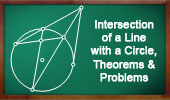# Plane Geometry: Intersection of a Line with a Circle, Secant, Theorems and Problems 2Problem 538. Triangle, Perpendicular Bisector, Circumcircle, Midpoint, Intersection of a Line with a Circle. Problem 532. Triangle, Circumcircle, Equal Angles, Perpendicular, Area, Intersection of a Line with a Circle. Problem 530. Cyclic Quadrilateral, Diagonal, Diameter, Perpendicular, Congruence, Intersection of a Line with a Circle. Problem 529. Right Trapezoid, Circle, Diameter, Intersection of a Line with a Circle. Problem 527. Circle, Tangent, Secant, Chord, Midpoint, Measurement, Intersection of a Line with a Circle. Problem 526. Equilateral Triangle, Circumcircle, Chord, Measurement, Intersection of a Line with a Circle. Problem 525. Circles, Diameter, Tangent, Radius, Congruence, Measurement, Intersection of a Line with a Circle. Problem 522. Right Triangle, Circle, Diameter, Tangent, Intersection of a Line with a Circle. Problem 500. Circle, Diameter, Chord, Perpendicular, Measure, Intersection of a Line with a Circle. Problem 493. Right Triangle, Circumcircle, Perpendicular, Chord, Intersection of a Line with a Circle.

Go to Page: Previous | 1 | 2 | 3 | 4 | 5 | Next

 Home | Search | Geometry | Circles | Post a comment | Email | By Antonio Gutierrez Last updated: Dec 12, 2015